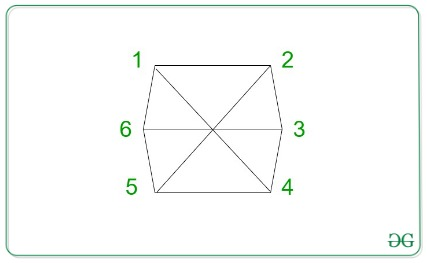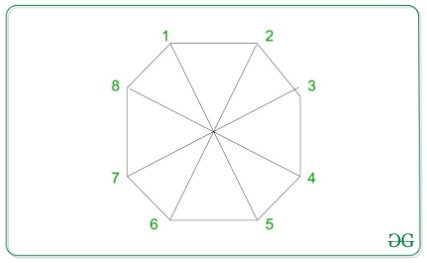Open in App
Not now

# Find the vertex diagonally opposite to the vertex M from an N-sided polygon

• Last Updated : 17 Mar, 2021

Given two integers N and M, the task is to find the vertex diagonally opposite to the Mth vertex of an N-sided polygon.

Examples:

Input: N = 6, M = 2
Output:
Explanation:It can be observed from the image above that the vertex opposite to vertex 5 is 2.

Input: N = 8, M = 5
Output:
Explanation:It can be observed from the image above that the vertex opposite to vertex 8 is 1.

Approach: The following two cases need to be considered to solve the given problem:

1. If M > N / 2: The vertex will always be M — (N / 2).
2. If M ≤ N / 2: The vertex will always be M + (N / 2).

Below is the implementation of the above approach:

## C++

 `// C++ program for the above approach` `#include ``using` `namespace` `std;` `// Function to return the``// required vertex``int` `getPosition(``int` `N, ``int` `M)``{` `    ``// Case 1:``    ``if` `(M > (N / 2)) {``        ``return` `(M - (N / 2));``    ``}` `    ``// Case 2:``    ``return` `(M + (N / 2));``}` `// Driver Code``int` `main()``{``    ``int` `N = 8, M = 5;``    ``cout << getPosition(N, M);` `    ``return` `0;``}`

## Java

 `// Java program for``// the above approach``class` `GFG{` `// Function to return the``// required vertex``static` `int` `getPosition(``int` `N,``                       ``int` `M)``{``  ``// Case 1:``  ``if` `(M > (N / ``2``))``  ``{``    ``return` `(M - (N / ``2``));``  ``}` `  ``// Case 2:``  ``return` `(M + (N / ``2``));``}` `// Driver Code``public` `static` `void` `main(String[] args)``{``  ``int` `N = ``8``, M = ``5``;``  ``System.out.print(getPosition(N, M));` `}``}` `// This code is contributed by Rajput-Ji`

## Python3

 `# Python3 program for the``# above approach` `# Function to return the``# required vertex``def` `getPosition(N, M):``    ` `  ``# Case 1:``  ``if` `(M > (N ``/``/` `2``)):``    ``return` `(M ``-` `(N ``/``/` `2``))``   ` `  ``# Case 2:``  ``return` `(M ``+` `(N ``/``/` `2``))``  ` `# Driver Code``N ``=` `8``M ``=` `5` `print``(getPosition(N, M))` `# This code is contributed by code_hunt`

## C#

 `// C# program for the above approach``using` `System;` `class` `GFG{` `// Function to return the``// required vertex``static` `int` `getPosition(``int` `N, ``int` `M)``{``    ` `    ``// Case 1:``    ``if` `(M > (N / 2))``    ``{``        ``return` `(M - (N / 2));``    ``}``    ` `    ``// Case 2:``    ``return` `(M + (N / 2));``}` `// Driver Code``public` `static` `void` `Main(String[] args)``{``    ``int` `N = 8, M = 5;``    ` `    ``Console.Write(getPosition(N, M));``}``}` `// This code is contributed by Amit Katiyar`

## Javascript

 ``

Output:

`1`

Time Complexity: O(1)
Auxiliary Space: O(1)

My Personal Notes arrow_drop_up